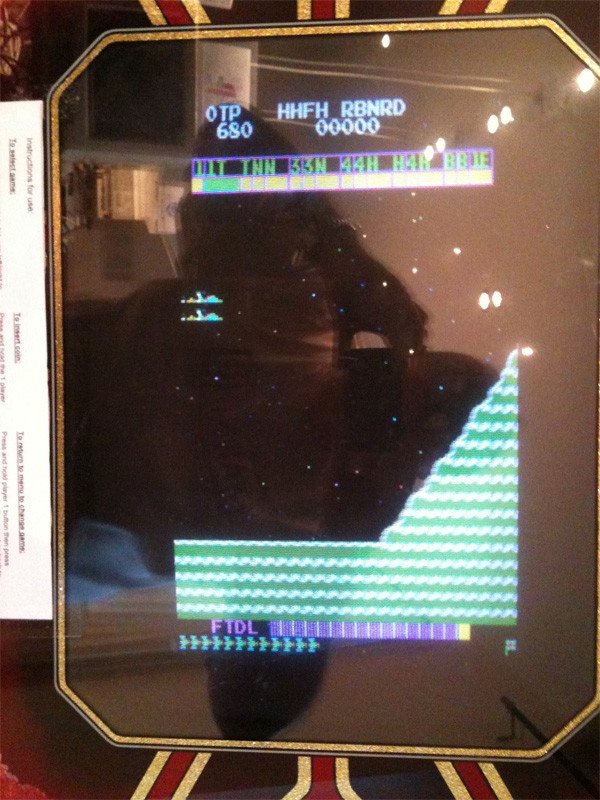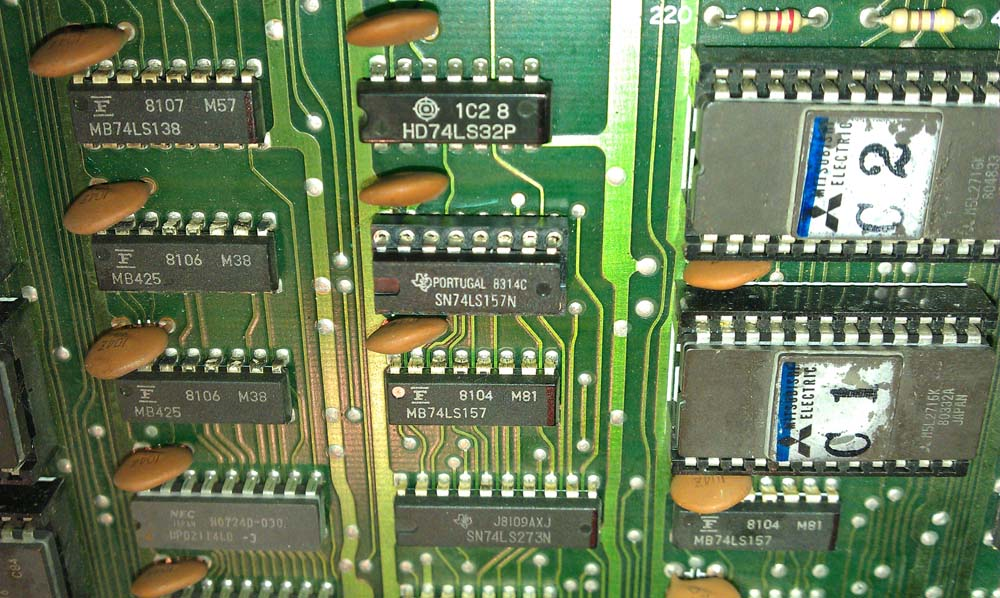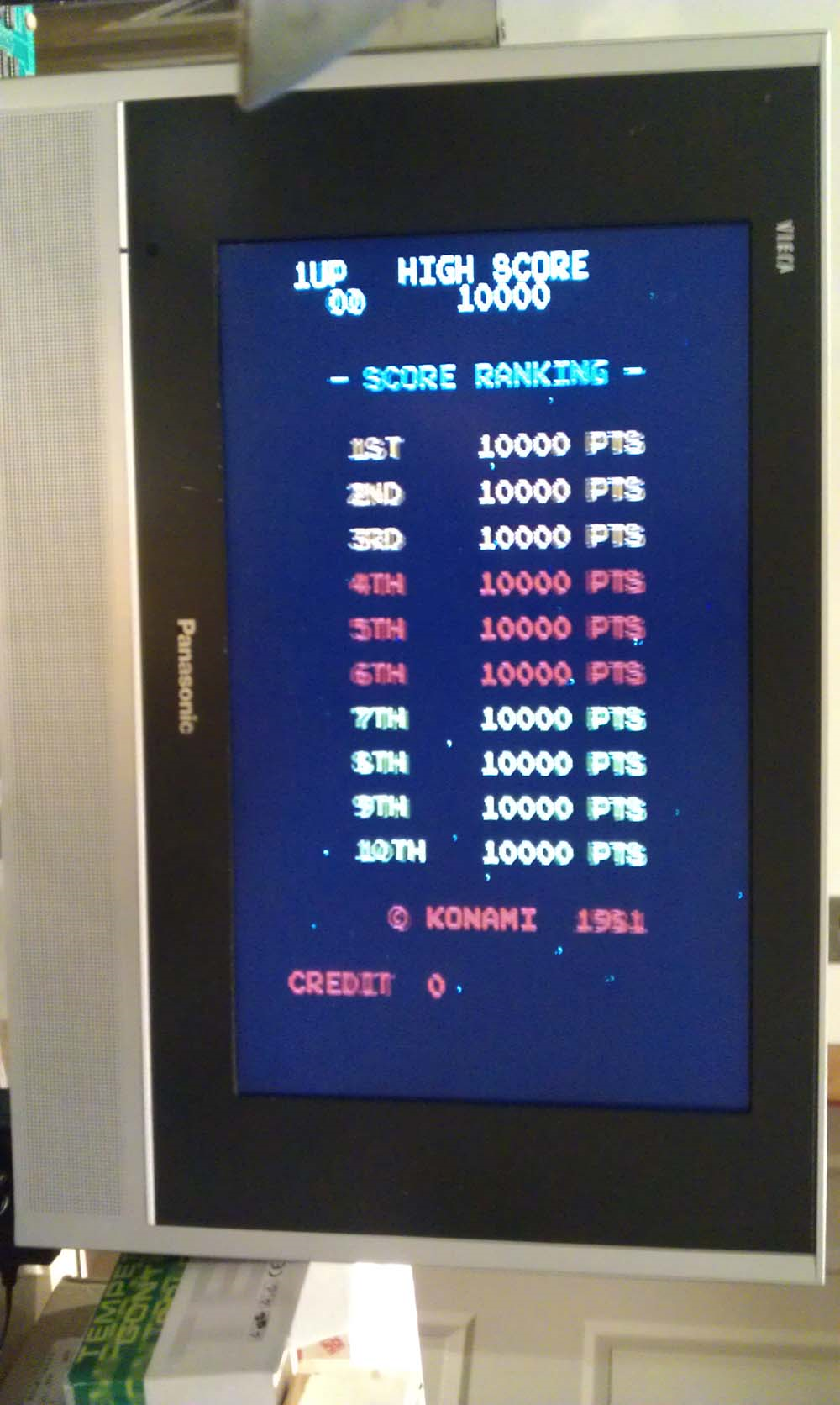Interesting one this, graphics were doubled up, wrong tiles used and the text was garbage;I quickly and correctly diagnosed that Bit 0 is stuck low on reads/writes from screen/object RAM.  You can tell by looking at the scoreline;

 00001000 = 08 = H … becomes 00001000 = 08 = H 00001001 = 09 = I … becomes 00001000 = 08 = H 00000111 = 07 = G … becomes 00000110 = 06 = F 00001000 = 08 = H … becomes 00001000 = 08 = H   00010011 = 19 = S … becomes 00010010 = 18 = R 00000011 = 03 = C … becomes 00000010 = 02 = B 00001111 = 15 = O … becomes 00001110 = 14 = N 00010010 = 18 = R … becomes 00010010 = 18 = R 00000101 = 05 = E … becomes 00000100 = 04 = D

I started to look at the video RAM addressing circuit, found a LS157 Multiplexer at 4G used to access a bank of video RAM with pin 9 stuck low.  Removed, fitted socket and new LS157;Now working 100%;Sorry, the comment form is closed at this time.